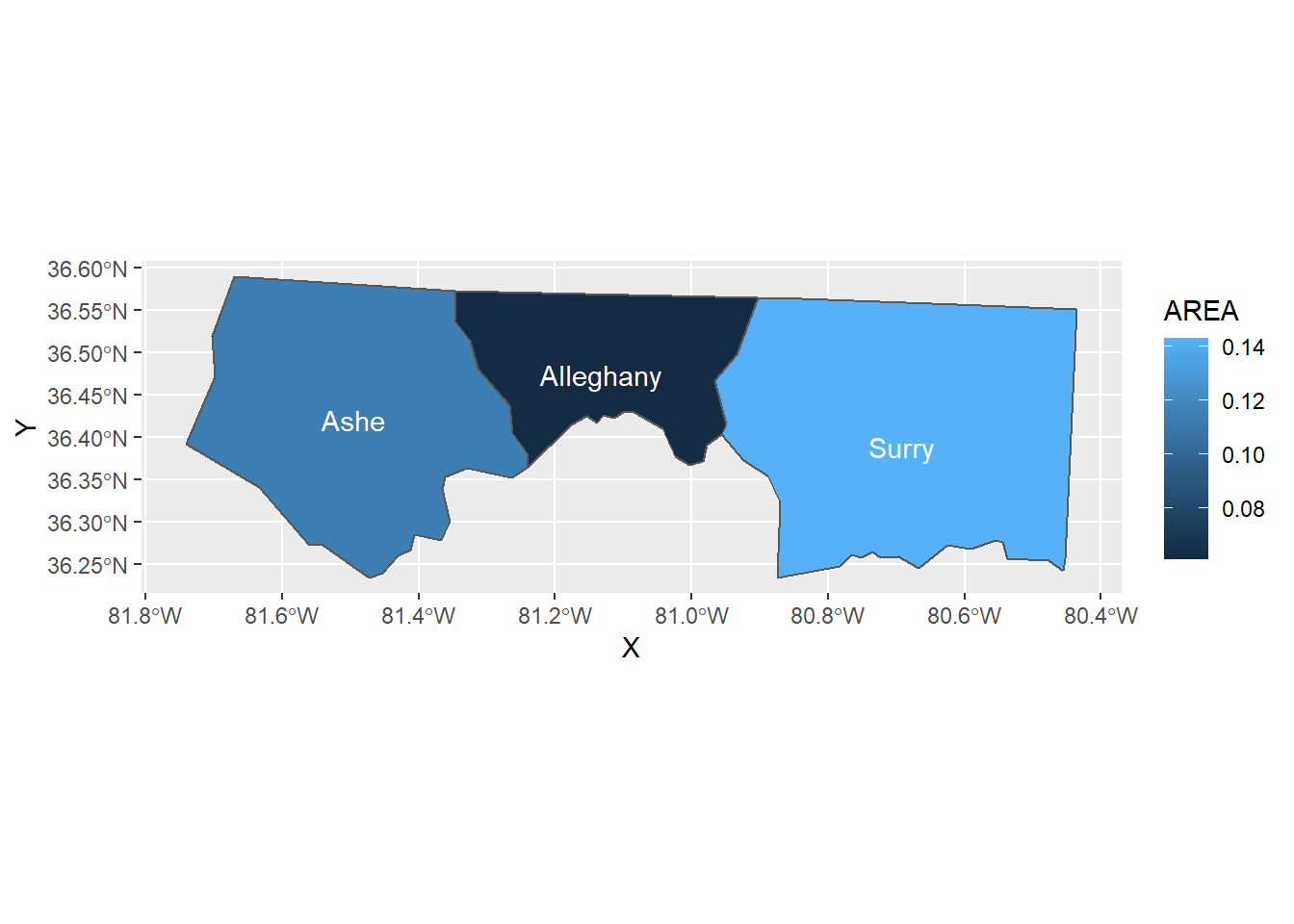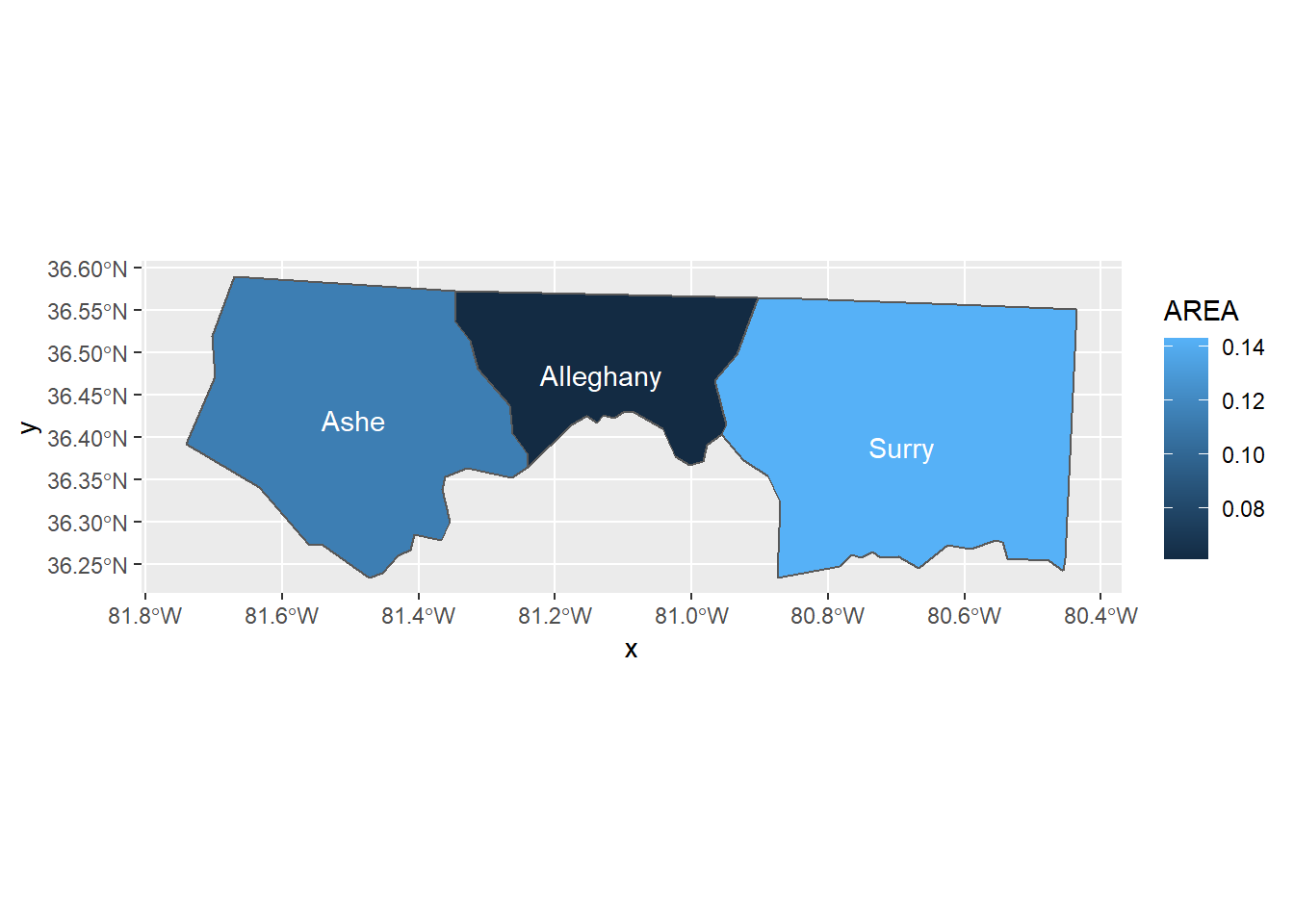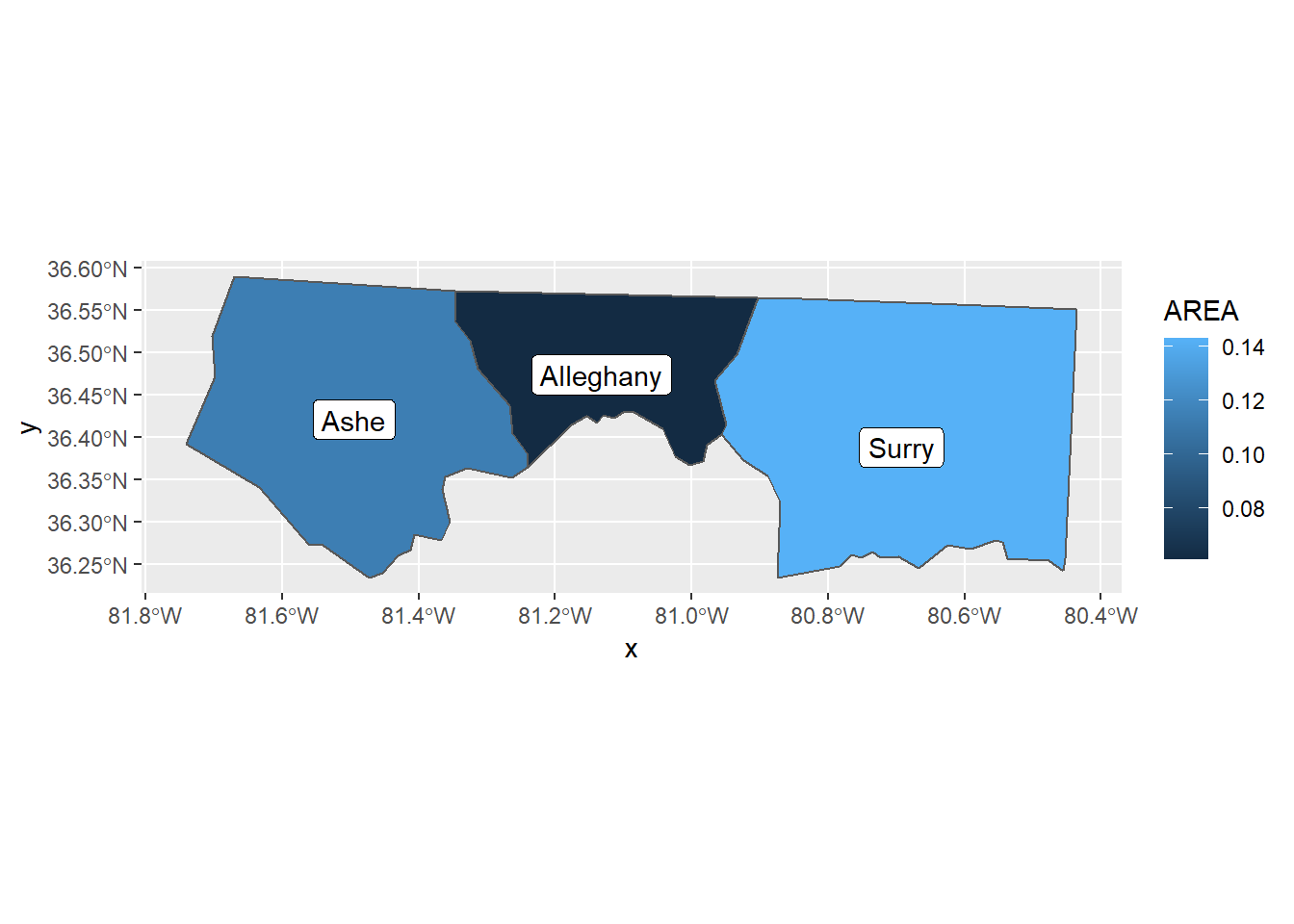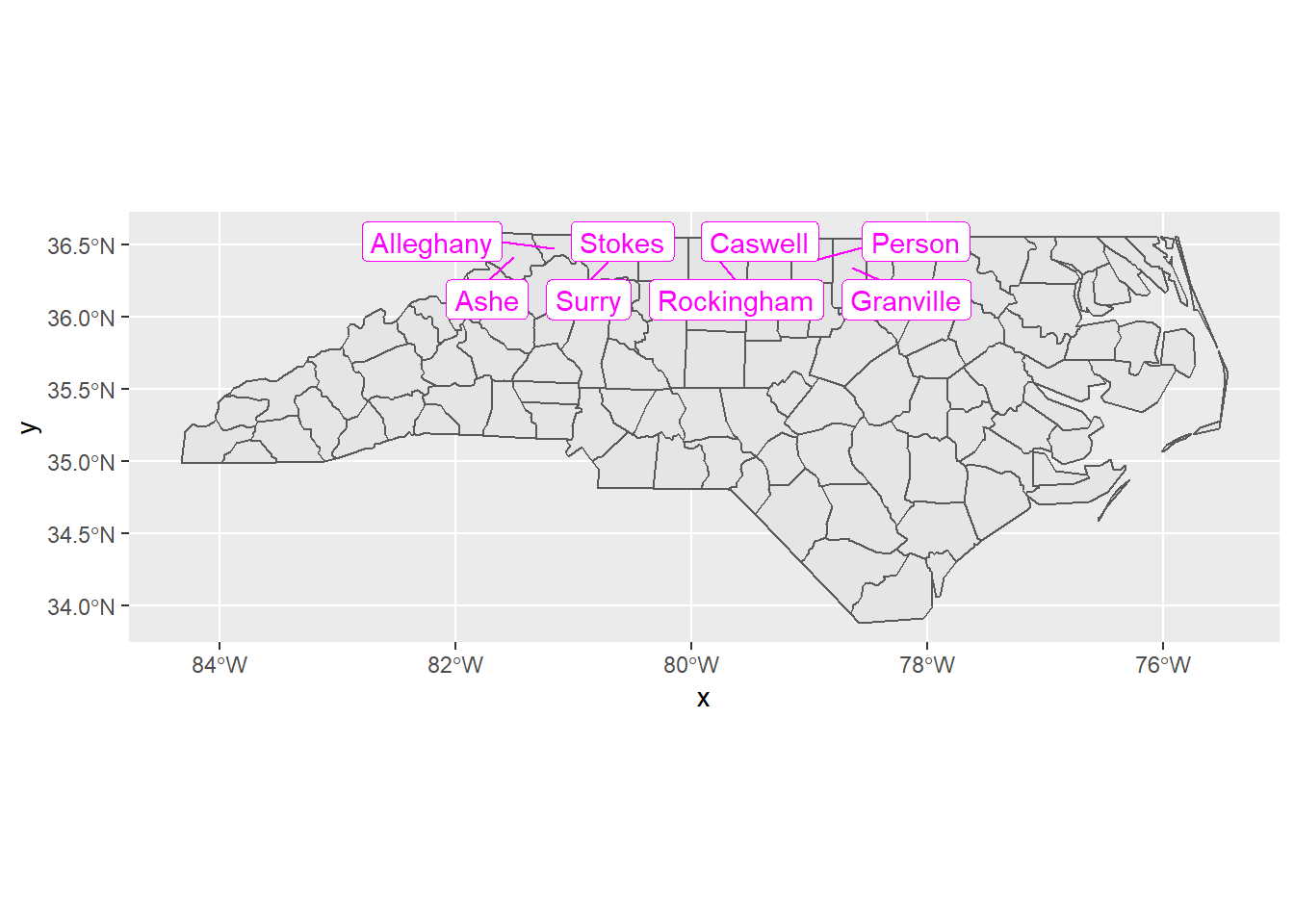# geom_sf_text() and geom_sf_label() Are Coming!

tidyverse
ggplot2
Author

Hiroaki Yutani

Published

October 10, 2018

ggplot2 v3.1.0 will be released soon (hopefully), so let me do a spoiler about a small feature I implemented, `geom_sf_label()` and `geom_sf_text()`.

## How can we add label/text with `geom_sf()`?

`geom_sf()` is one of the most exciting features introduced in ggplot2 v3.0.0. It magically allows us to plot sf objects according to their geometries’ shapes (polygons, lines and points).

But, for plotting them as some other shapes than the original ones, we cannot rely on `geom_sf()` so it needs a bit of data transformation beforehand. Suppose we want to add text on each geometry, we need to

1. calculate the proper point to add text/labels per geometry by some function like `sf::st_centroid()` and `sf::st_point_on_surface()`,
2. retrieve the coordinates from the calculated points by `sf::st_coordinates()`, and
3. use `geom_text()` or `geom_label()` with the coordinates

The code for this would be like below:

``````library(ggplot2)

nc <- sf::st_read(system.file("shape/nc.shp", package = "sf"), quiet = TRUE)

# use only first three elements
nc3 <- nc[1:3, ]

# choose a point on the surface of each geometry
nc3_points <- sf::st_point_on_surface(nc3)``````
``````Warning in st_point_on_surface.sf(nc3): st_point_on_surface assumes attributes
are constant over geometries of x``````
``````Warning in st_point_on_surface.sfc(st_geometry(x)): st_point_on_surface may not
give correct results for longitude/latitude data``````
``````# retrieve the coordinates
nc3_coords <- as.data.frame(sf::st_coordinates(nc3_points))
nc3_coords\$NAME <- nc3\$NAME

nc3_coords``````
``````          X        Y      NAME
1 -81.49496 36.42112      Ashe
2 -81.13241 36.47396 Alleghany
3 -80.69280 36.38828     Surry``````
``````ggplot() +
geom_sf(data = nc3, aes(fill = AREA)) +
geom_text(data = nc3_coords, aes(X, Y, label = NAME), colour = "white")``````Phew, this seems not so difficult, but I feel the code is a bit too long…

## `geom_sf_label()` and `geom_sf_text()`

For this purpose, upcoming ggplot2 v3.1.0 provides two new geoms, `geom_sf_text()` and `geom_sf_label()`. The code equivalent to above can be written as:

``````# texts and labels
p <- ggplot(nc3) +
geom_sf(aes(fill = AREA))

p + geom_sf_text(aes(label = NAME), colour = "white")``````
``````Warning in st_point_on_surface.sfc(sf::st_zm(x)): st_point_on_surface may not
give correct results for longitude/latitude data``````For labels, use `geom_sf_label()`:

``p + geom_sf_label(aes(label = NAME))``
``````Warning in st_point_on_surface.sfc(sf::st_zm(x)): st_point_on_surface may not
give correct results for longitude/latitude data``````## Protip: `stat_sf_coordinates()`

Under the hood, a `Stat` called `stat_sf_coordinates()` does the necessary calculations. If you are an expert of ggplot2, please play with this by combining with other `Geom`s. As an example, here’s a preliminary version of `geom_sf_label_repel()` (which I want to implement next…):

``````ggplot(nc) +
geom_sf() +
ggrepel::geom_label_repel(
data = nc[c(1:3, 10:14), ],
aes(label = NAME, geometry = geometry),
stat = "sf_coordinates",
min.segment.length = 0,
colour = "magenta",
segment.colour = "magenta"
)``````
``````Warning in st_point_on_surface.sfc(sf::st_zm(x)): st_point_on_surface may not
give correct results for longitude/latitude data``````For other cool NEWS of ggplot2 v3.1.0, please read the `NEWS.md` :)